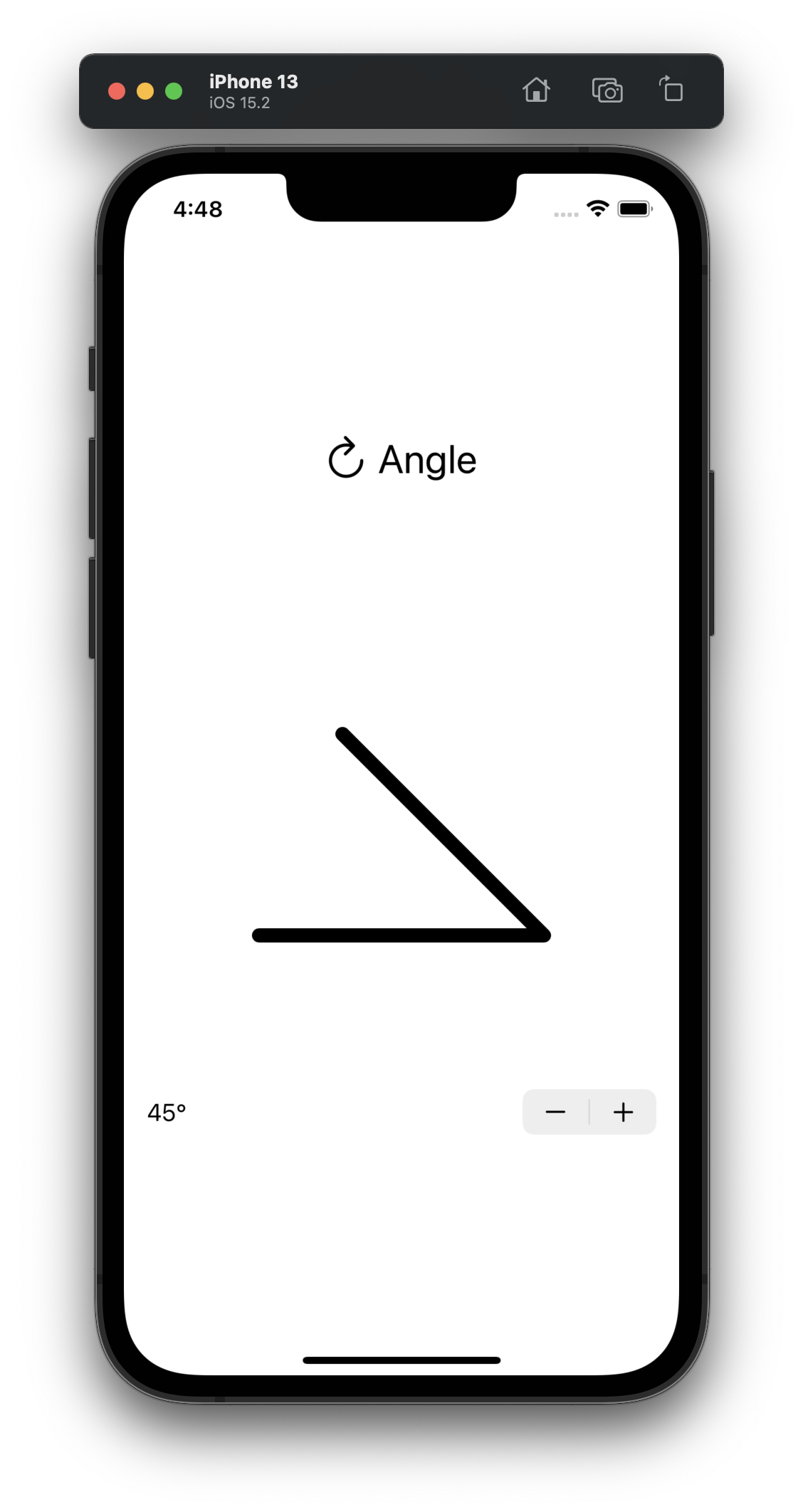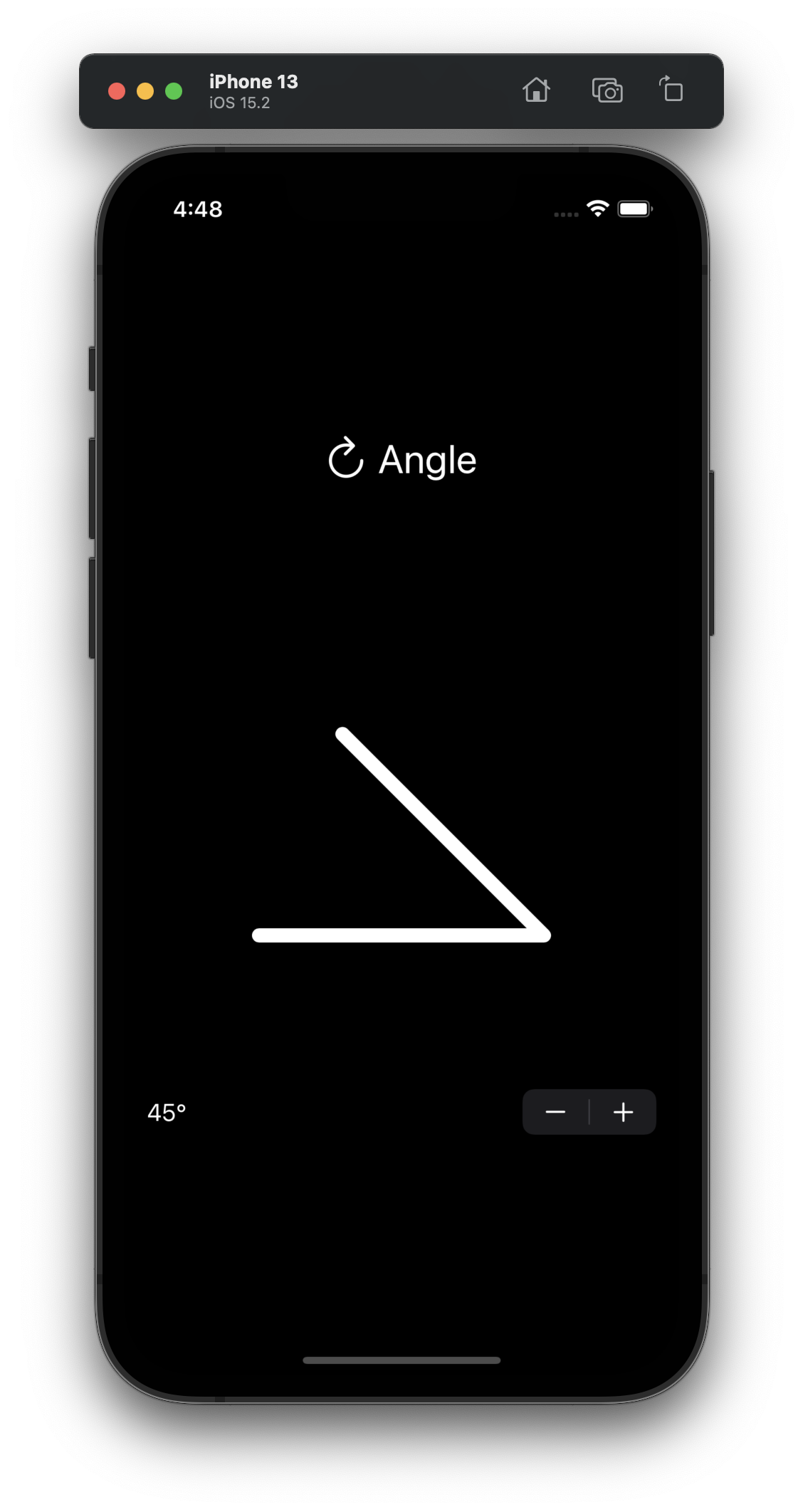# Aim

The idea for this first step is quite simple: draw a shape consisting of two lines on screen representing an angle between 0 and 90 degrees.# Setup

Above the shape I’ll add a label called “Angle” and use the `arrow.clockwise` symbol from SF Symbols. Below the shape a `Stepper` control displays the current angle. For now it suffices to store the angle in an `@State` var. Later on this can be exposed as an `@Binding`.

``````struct AngleView: View {

@State var angle: CGFloat = 45

var body: some View {
VStack {
Label("Angle", systemImage: "arrow.clockwise")
AngleShape(angle: angle)
Stepper("\(Int(round(angle)))°", value: \$angle, step: 5)
}
}
}
``````

# Custom Shape

When the angle is changed through the stepper, I want to animate the change. To let SwiftUI know how to change the shape between two angles a new struct conforming to the `Shape` protocol is needed. This is done in the method `func path(in rect: CGRect) -> Path`. The `Shape` protocol in turn conforms to the `Animatable` protocol, which adds a `var animatableData` to the struct.

So I create a new struct called AngleShape. For this shape just the angle is enough to be able create a path for all values between two angles, so the `animatableData` is a `CGFloat`.

``````struct AngleShape: Shape {
var angle: CGFloat

var animatableData: CGFloat {
get { angle }
set { angle = newValue }
}

func path(in rect: CGRect) -> Path {
// …
}
}
``````

# Trigonometry

It looks better if there is some additional space around the angle, so I am adding edge insets. The calculation of the first two points is then simple enough after insetting the passed in rect. The third point can be calculated with some trigonometry. Here paper and pencil are still essential tools for developers! Just be sure that the used rect is square so the hypotenuse is always the same lenght.

``````    var insets: UIEdgeInsets = .init(top: 100, left: 100, bottom: 100, right: 100)

func path(in rect: CGRect) -> Path {
let insetRect = rect.inset(by: insets)
assert(insetRect.width == insetRect.height, "Rect must be square")

var path = Path()
path.move(to: CGPoint(x: insetRect.minX, y: insetRect.maxY))

let hypotenuse: CGFloat = insetRect.maxX - insetRect.minX
let opposite = hypotenuse * sin(angleInRadians)
let x = insetRect.minX + hypotenuse - adjacent
let y = insetRect.minY + hypotenuse - opposite

return path
}
``````

In the above calculation the angle is given in degrees, since that’s easiest for the user, but to use it with Swift it needs to converted to radians. This is done by adding an extension on `CGFloat`.

``````extension CGFloat {
self * 360 / (.pi * 2)
}

self * .pi * 2 / 360
}
}
``````

# Drawing Style

The AngleShape is now ready to be used. I used a somewhat abritrary frame of 400 by 400 pixels. A good choice of color is `.foreground` so that it looks as expected in both light and dark mode. For the `StrokeStyle` use rounded line caps and line joins to avoid the issue where the pointy bit of the angle becomes long or gets replaced by a bevel. Lastly add `.animation(.default, value: angle)` to let SwiftUI animate the angle changes.

``````struct AngleView: View {

@State var angle: CGFloat = 45

var body: some View {
VStack {
Label("Angle", systemImage: "arrow.clockwise")
.font(.title)
AngleShape(angle: angle)
.stroke(.foreground,  style: StrokeStyle(lineWidth: 10, lineCap: .round, lineJoin: .round))
.frame(width: 400, height: 400)
.animation(.default, value: angle)
Stepper("\(Int(round(angle)))°", value: \$angle, step: 5)
}
}
}
``````

# Code

You can find all the code created in this post on GitHub.